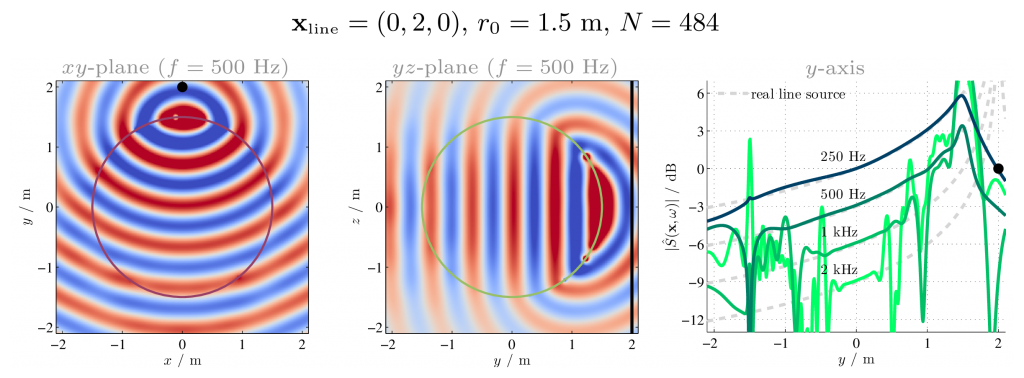# Paper: Sound Field Synthesis of Virtual Cylindrical Waves using Circular and Spherical Loudspeaker Arrays

The paper Sound Field Synthesis of Virtual Cylindrical Waves using Circular and Spherical Loudspeaker Arrays was presented at the 138th Convention of the Audio Engineering Society in Warsaw, Poland. The presentation slides and the MATLAB script used for the simulations are available for download. The latter has to be used with the SFS toolbox.Eq. (19) $$D_\text{3D}(\alpha_0,\beta_0,\omega) = \frac{2}{{r_0}^2} \sum_{n=0}^{\infty} \sum_{m=-n}^{n} \frac{\color{RoyalBlue}{i^{m-n+1}} \mathring{S}_{m}(\omega)}{\tfrac{\omega}{c} h_{n}^{(2)}(\tfrac{\omega}{c}r_0)} Y_{n}^{m}(\tfrac{\pi}{2},0)^\ast Y_{n}^{m}(\beta_0,\alpha_0)$$ Eq. (20) $$D_\text{3D,ls}(\alpha_0,\beta_0,\omega) = \frac{1}{2{r_0}^2} \sum_{n=0}^{\infty} \sum_{m=-n}^{n} \frac{\color{RoyalBlue}{i^{m-n}} H_{m}^{(2)}(\frac{\omega}{c}r_\text{ls})}{\tfrac{\omega}{c} h_{n}^{(2)}(\tfrac{\omega}{c}r_0)} Y_{n}^{m}(\tfrac{\pi}{2},\alpha_\text{ls})^\ast Y_{n}^{m}(\beta_0,\alpha_0)$$
This entry was posted in Publications. Bookmark the permalink.

This site uses Akismet to reduce spam. Learn how your comment data is processed.